Anúncio

# RF Circuit Design - [Ch2-1] Resonator and Impedance Matching

13 de Jun de 2015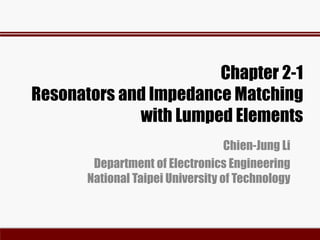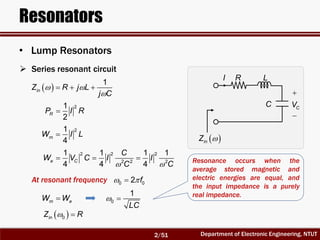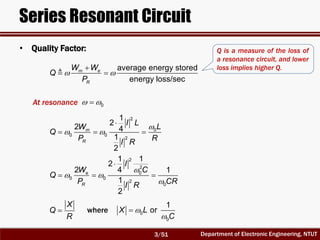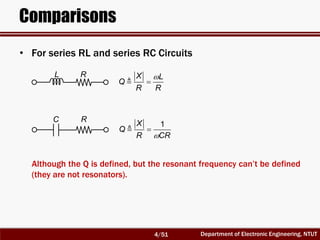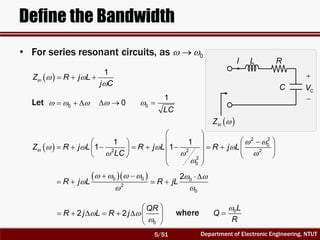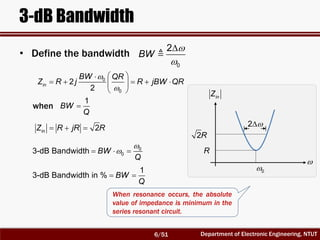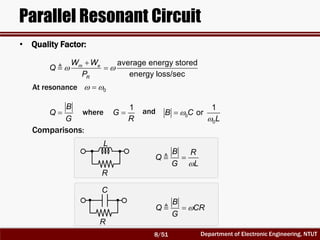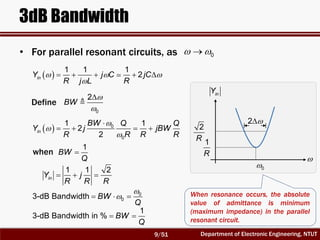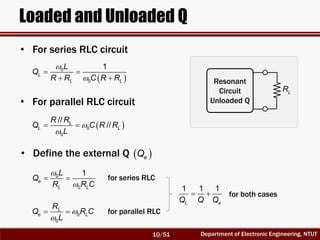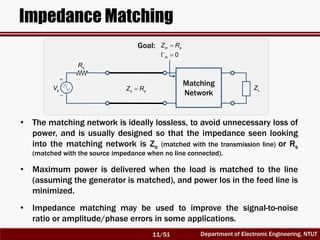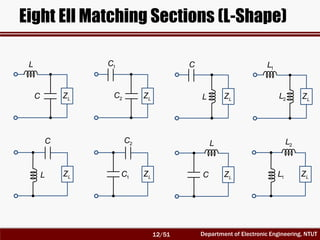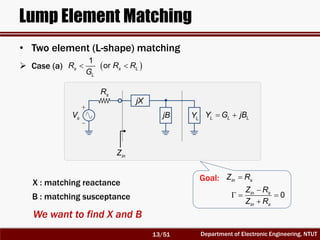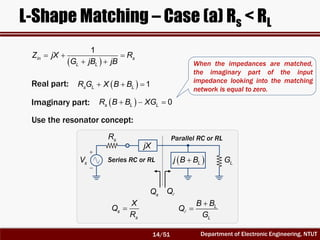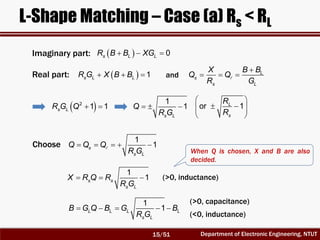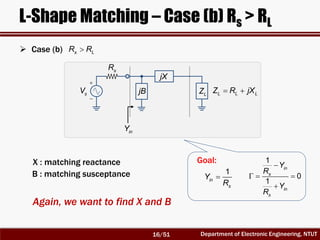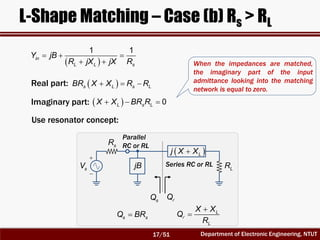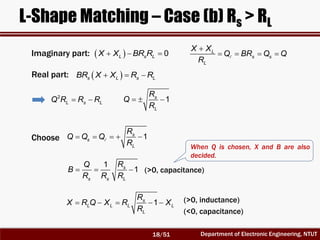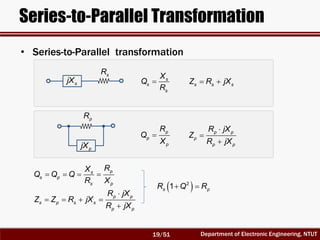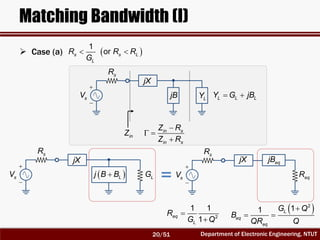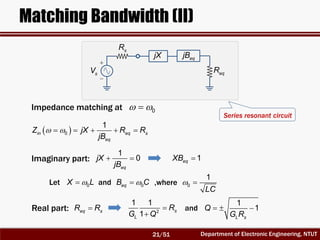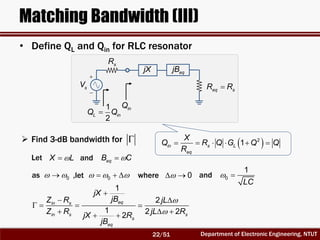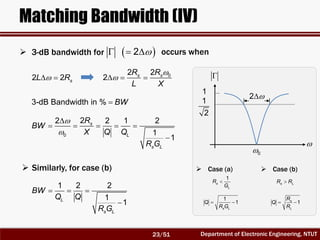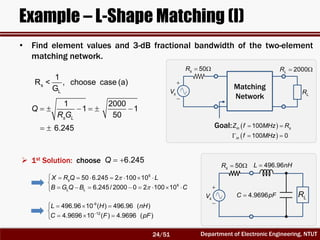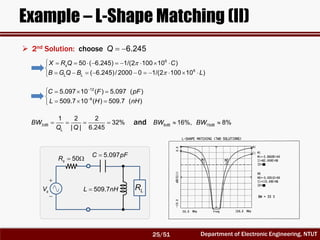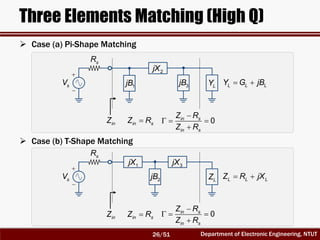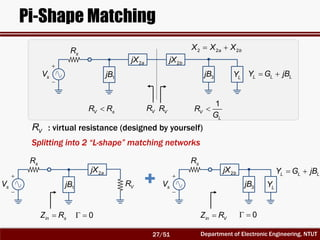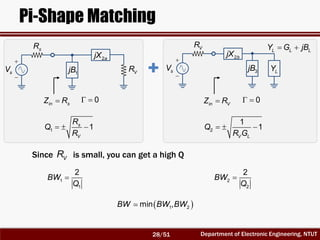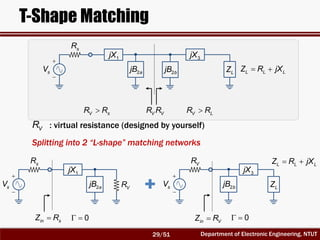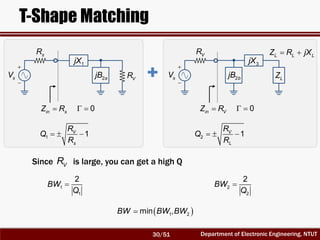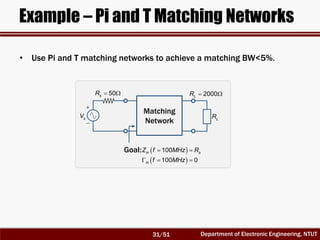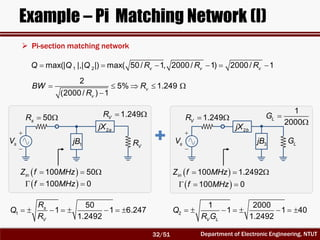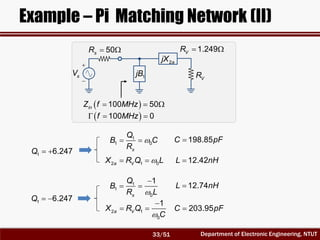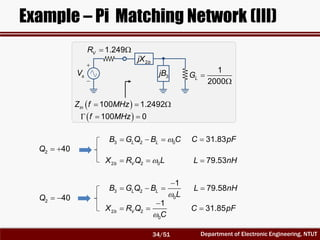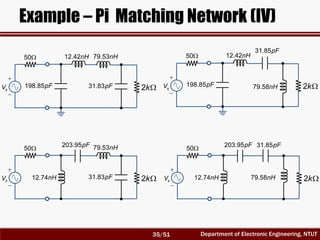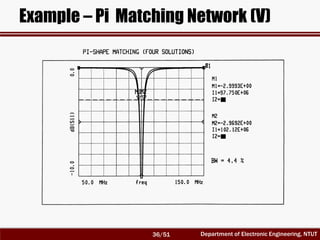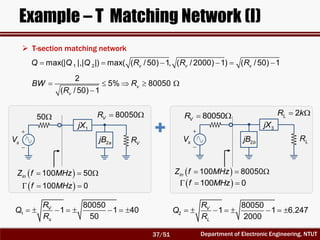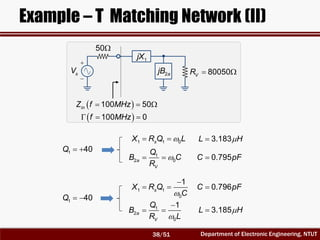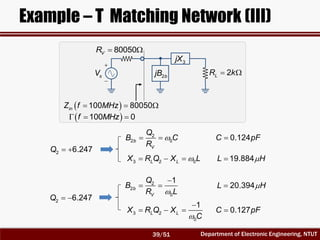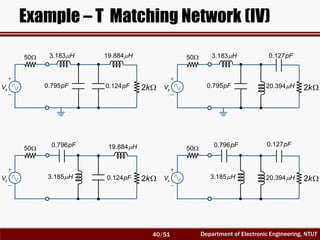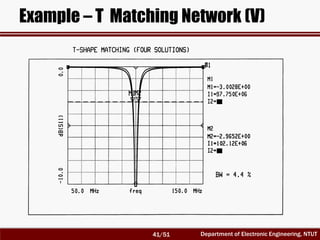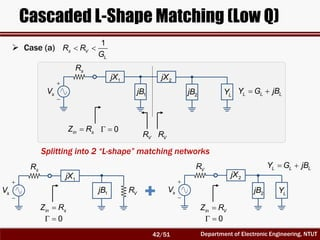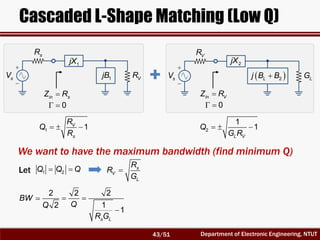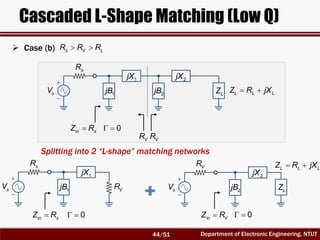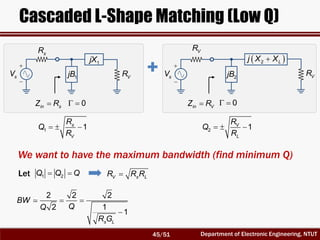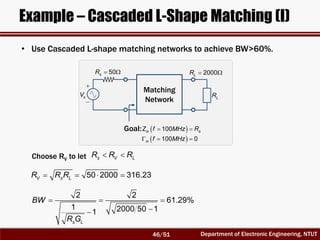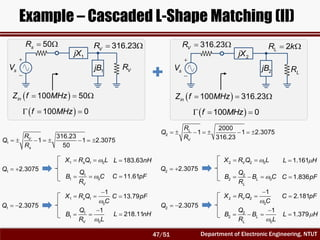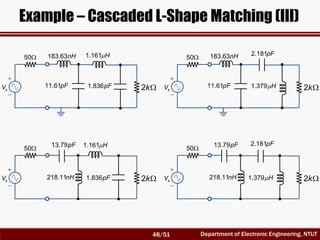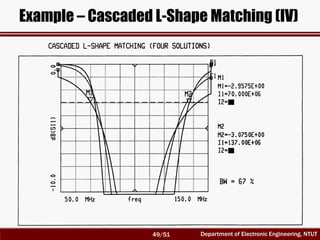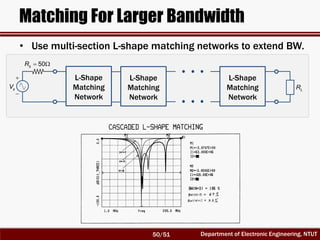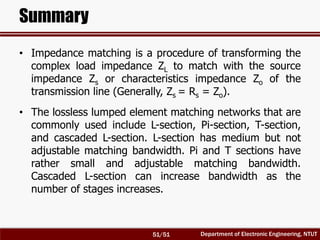1 de 51
Anúncio

### RF Circuit Design - [Ch2-1] Resonator and Impedance Matching

1. Chapter 2-1 Resonators and Impedance Matching with Lumped Elements Chien-Jung Li Department of Electronics Engineering National Taipei University of Technology
2. Department of Electronic Engineering, NTUT Resonators • Lump Resonators  Series resonant circuit I R L C  inZ CV          1 inZ R j L j C  21 2 RP I R  21 4 mW I L      2 2 2 2 2 2 1 1 1 1 4 4 4 e C C W V C I I C C At resonant frequency  0 02 f m eW W  0 1 LC   0inZ R Resonance occurs when the average stored magnetic and electric energies are equal, and the input impedance is a purely real impedance. 2/51
3. Department of Electronic Engineering, NTUT Series Resonant Circuit • Quality Factor:     average energy stored energy loss/sec m e R W W Q P At resonance   0        2 0 0 0 2 1 22 4 1 2 m R I L LW Q P RI R         2 2 0 0 0 2 0 1 1 2 2 4 1 1 2 e R I W C Q P CRI R  X Q R where    0 0 1 orX L C Q is a measure of the loss of a resonance circuit, and lower loss implies higher Q. 3/51
4. Department of Electronic Engineering, NTUT Comparisons   X L Q R R L R C R   1X Q R CR Although the Q is defined, but the resonant frequency can’t be defined (they are not resonators). • For series RL and series RC Circuits 4/51
5. Department of Electronic Engineering, NTUT Define the Bandwidth • For series resonant circuits, as   0        1 inZ R j L j C     0 Let   0  0 1 LC                                      2 2 0 22 2 2 0 1 1 1 1inZ R j L R j L R j L LC                  0 0 0 2 0 2 R j L R jL               0 2 2 QR R j L R j   0L Q R I L R C  inZ CV   where 5/51
6. Department of Electronic Engineering, NTUT 3-dB Bandwidth    0 2 BW             0 0 2 2 in BW QR Z R j R jBW QR when  1 BW Q    2inZ R jR R     0 03-dB Bandwidth BW Q   1 3-dB Bandwidth in % BW Q inZ 0  R 2R 2 • Define the bandwidth When resonance occurs, the absolute value of impedance is minimum in the series resonant circuit. 6/51
7. Department of Electronic Engineering, NTUT Parallel Resonant Circuit  Parallel resonant circuit        1 1 inY j C R j L  2 2 R V P R  21 4 eW V C      2 2 2 2 2 2 1 1 1 4 4 4 m L V V W I L L L L At resonance, the angular resonant frequency  0 02 f m eW W  0 1 LC   0 1 inY R LI LR C  inY V   7/51
8. Department of Electronic Engineering, NTUT Parallel Resonant Circuit • Quality Factor:     average energy stored energy loss/sec m e R W W Q P At resonance   0  B Q G where    0 0 1 orB C L  1 G R Comparisons:   B R Q G L  B Q CR G L R C R and 8/51
9. Department of Electronic Engineering, NTUT 3dB Bandwidth • For parallel resonant circuits, as   0           1 1 1 2inY j C jC R j L R    0 2 BWDefine          0 0 1 1 2 2 in BW Q Q Y j jBW R R R R when  1 BW Q    1 1 2 inY j R R R     0 03-dB Bandwidth BW Q   1 3-dB Bandwidth in % BW Q inY 0  1 R 2 R 2 When resonance occurs, the absolute value of admittance is minimum (maximum impedance) in the parallel resonant circuit. 9/51
10. Department of Electronic Engineering, NTUT Loaded and Unloaded Q LR Resonant Circuit Unloaded Q • For series RLC circuit • For parallel RLC circuit         0 0 1 L L L L Q R R C R R      0 0 // //L L L R R Q C R R L • Define the external Q  eQ    0 0 1 e L L L Q R R C for series RLC     0 0 L e L R Q R C L   1 1 1 L eQ Q Q for parallel RLC for both cases 10/51
11. Department of Electronic Engineering, NTUT Impedance Matching Matching Network in sZ R   sV sR LZo sZ R   0in Goal: • The matching network is ideally lossless, to avoid unnecessary loss of power, and is usually designed so that the impedance seen looking into the matching network is Zo (matched with the transmission line) or Rs (matched with the source impedance when no line connected). • Maximum power is delivered when the load is matched to the line (assuming the generator is matched), and power los in the feed line is minimized. • Impedance matching may be used to improve the signal-to-noise ratio or amplitude/phase errors in some applications. 11/51
12. Department of Electronic Engineering, NTUT Eight Ell Matching Sections (L-Shape) LZ1C 2C LZL C LZ1L 2L LZC L LZC L LZ2C 1C LZL C LZ2L 1L 12/51
13. Department of Electronic Engineering, NTUT Lump Element Matching • Two element (L-shape) matching   sV sR jX jB LY  L L LY G jB inZ X : matching reactance B : matching susceptance We want to find X and B  Case (a)    1 ors s L L R R R G in sZ R      0in s in s Z R Z R Goal: 13/51
14. Department of Electronic Engineering, NTUT L-Shape Matching – Case (a) Rs < RL        1 in s L L Z jX R G jB jB Real part:     1s L LR G X B B Imaginary part:     0s L LR B B XG   sV sR jX   Lj B B LG lQsQ Use the resonator concept: Series RC or RL Parallel RC or RL s s X Q R l   L L B B Q G When the impedances are matched, the imaginary part of the input impedance looking into the matching network is equal to zero. 14/51
15. Department of Electronic Engineering, NTUT L-Shape Matching – Case (a) Rs < RL Imaginary part:     0s L LR B B XG     L s s L X B B Q Q R G lReal part:     1s L LR G X B B   2 1 1s LR G Q    1 1 s L Q R G Choose l     1 1s s L Q Q Q R G    1 1s s s L X R Q R R G and      1 1L L L L s L B G Q B G B R G (>0, capacitance) (<0, inductance) (>0, inductance)         or 1L s R R When Q is chosen, X and B are also decided. 15/51
16. Department of Electronic Engineering, NTUT L-Shape Matching – Case (b) Rs > RL   sV sR jX jB LZ  L L LZ R jX inY X : matching reactance B : matching susceptance  1 in s Y R      1 0 1 in s in s Y R Y R Again, we want to find X and B  Case (b) s LR R Goal: 16/51
17. Department of Electronic Engineering, NTUT L-Shape Matching – Case (b) Rs > RL        1 1 in L L s Y jB R jX jX R Real part:    s L s LBR X X R R Imaginary part:     0L s LX X BR R Use resonator concept: s sQ BR l   L L X X Q R   sV sR   Lj X X jB LR lQsQ Parallel RC or RL Series RC or RL When the impedances are matched, the imaginary part of the input admittance looking into the matching network is equal to zero. 17/51
18. Department of Electronic Engineering, NTUT L-Shape Matching – Case (b) Rs > RL Imaginary part: l     L s s L X X Q BR Q Q R Real part:  2 L s LQ R R R   1s L R Q R Choose l    1s s L R Q Q Q R     1s L L L L L R X R Q X R X R (>0, inductance)    1 1s s s L RQ B R R R (>0, capacitance) (<0, capacitance)     0L s LX X BR R    s L s LBR X X R R When Q is chosen, X and B are also decided. 18/51
19. Department of Electronic Engineering, NTUT Series-to-Parallel Transformation • Series-to-Parallel transformation sR sjX pR pjX  s s s X Q R  s s sZ R jX  p p p R Q X    p p p p p R jX Z R jX     ps s p s p RX Q Q Q R X       p p s p s s p p R jX Z Z R jX R jX   2 1s pR Q R 19/51
20. Department of Electronic Engineering, NTUT Matching Bandwidth (I)  Case (a)    1 ors s L L R R R G   sV sR jX jB LY  L L LY G jB inZ     in s in s Z R Z R   sV sR jX   Lj B B LG   sV sR jX eqjB eqR   2 1 1 1 eq L R G Q  2 11 L eq eq G Q B QR Q    20/51
21. Department of Electronic Engineering, NTUT Matching Bandwidth (II)   sV sR jX eqjB eqR Impedance matching at   0       0 1 in eq s eq Z jX R R jB Imaginary part:   1 0 eq jX jB  1eqXB Let  0X L  0eqB C  0 1 LC Real part: eq sR R   2 1 1 1 s L R G Q    1 1 L s Q G R and ,where and Series resonant circuit 21/51
22. Department of Electronic Engineering, NTUT Matching Bandwidth (III)   sV sR jX eqjB eq sR R inQ  1 2 L inQ Q • Define QL and Qin for RLC resonator       2 1in s L eq X Q R Q G Q Q R  Find 3-dB bandwidth for  Let X L eqB C as   0 ,let     0  0 1 LC   0             1 2 1 2 22 eqin s in s s s eq jX jBZ R jL Z R jL RjX R jB and where and 22/51
23. Department of Electronic Engineering, NTUT Matching Bandwidth (IV)  3-dB bandwidth for    2 occurs when  2 2 sL R     02 2 2 s sR R L X 3-dB Bandwidth in % BW         0 22 2 1 2 1 1 s L s L R BW X Q Q R G  Similarly, for case (b)     1 2 2 1 1L s L BW Q Q R G  0  1 2 1 2   1 1 s L Q R G  Case (a)  1 s L R G  1s L R Q R  Case (b) s LR R 23/51
24. Department of Electronic Engineering, NTUT Example – L-Shape Matching (I) • Find element values and 3-dB fractional bandwidth of the two-element matching network.       1 2000 1 1 50s L Q R G s L 1 R < , choose case (a) G  6.245Q                    6 6 50 6.245 2 100 10 6.245/ 2000 0 2 100 10 s L L X R Q L B G Q B C  1st Solution: choose Matching Network   100in sZ f MHz R   sV  50sR  2000LR    100 0in f MHz Goal: LR LR 4.9696C pF  496.96L nH   sV  50sR          -9 12 496.96 10 ( ) 496.96 ( ) 4.9696 10 ( ) 4.9696 ( ) L H nH C F pF   6.245 24/51
25. Department of Electronic Engineering, NTUT Example – L-Shape Matching (II)                        6 6 50 ( 6.245) 1/(2 100 10 ) ( 6.245)/ 2000 0 1/(2 100 10 ) s L L X R Q C B G Q B L  6.245Q 2nd Solution: choose           12 9 5.097 10 ( ) 5.097 ( ) 509.7 10 ( ) 509.7 ( ) C F pF L H nH LR   sV  50sR  509.7L nH  5.097C pF    3dB 1 2 2 32% | | 6.245L BW Q Q  9dB 15dB16%, 8%BW BWand 25/51
26. Department of Electronic Engineering, NTUT Three Elements Matching (High Q)  Case (a) Pi-Shape Matching   sV sR 2jX 3jB LY  L L LY G jB inZ in sZ R      0in s in s Z R Z R 1jB   sV sR 1jX LZ  L L LZ R jX inZ in sZ R      0in s in s Z R Z R 2jB 3jX  Case (b) T-Shape Matching 26/51
27. Department of Electronic Engineering, NTUT Pi-Shape Matching   sV sR 2ajX 3jB LY  L L LY G jB VR 1jB 2bjX VR  2 2 2a bX X X VR : virtual resistance (designed by yourself)  1 V L R G V sR R Splitting into 2 “L-shape” matching networks   sV sR 2ajX 3jB LY  L L LY G jB 1jB 2bjX VR   sV sR in sZ R   0 in VZ R   0 27/51
28. Department of Electronic Engineering, NTUT Pi-Shape Matching   sV sR 2ajX 3jB LY  L L LY G jB 1jB 2bjX VR   sV VR in sZ R   0 in VZ R   0   1 1s V R Q R   2 1 1 V L Q R G Since is small, you can get a high QVR 1 1 2 BW Q 2 2 2 BW Q  1 2min ,BW BW BW 28/51
29. Department of Electronic Engineering, NTUT T-Shape Matching VR : virtual resistance (designed by yourself)   sV sR 1jX LZ  L L LZ R jX VR 2ajB 3jX 2bjB VR V LR RV sR R   sV sR 1jX LZ  L L LZ R jX in sZ R   0 2ajB 3jX VR 2bjB   sV VR in VZ R   0 Splitting into 2 “L-shape” matching networks 29/51
30. Department of Electronic Engineering, NTUT T-Shape Matching   sV sR 1jX in sZ R   0 2ajB VR LZ  L L LZ R jX 3jX 2bjB   sV VR in VZ R   0   1 1V s R Q R   2 1V L R Q R Since is large, you can get a high QVR 1 1 2 BW Q 2 2 2 BW Q  1 2min ,BW BW BW 30/51
31. Department of Electronic Engineering, NTUT Example – Pi and T Matching Networks • Use Pi and T matching networks to achieve a matching BW<5%. Matching Network   100in sZ f MHz R   sV  50sR  2000LR    100 0in f MHz Goal: LR 31/51
32. Department of Electronic Engineering, NTUT Example – Pi Matching Network (I)   sV  50sR 2ajX 3jB1jB 2bjX  1.249VR   sV  1.249VR    100 50inZ f MHz    100 0f MHz    100 1.2492inZ f MHz    100 0f MHz VR LG        1 50 1 1 6.247 1.2492 s V R Q R        2 1 2000 1 1 40 1.2492V L Q R G   1 2000 LG  Pi-section matching network      1 2max(| |,| |) max( 50/ 1, 2000/ 1) 2000/ 1v v vQ Q Q R R R       2 5% 1.249 (2000/ ) 1 v v BW R R 32/51
33. Department of Electronic Engineering, NTUT Example – Pi Matching Network (II)   sV  50sR 2ajX 1jB  1.249VR    100 50inZ f MHz    100 0f MHz VR  1 6.247Q  1 1 0 s Q B C R  198.85C pF  2 1 0a VX R Q L  12.42L nH  1 6.247Q    1 1 0 1 s Q B R L  203.95C pF    2 1 0 1 a VX R Q C  12.74L nH 33/51
34. Department of Electronic Engineering, NTUT Example – Pi Matching Network (III) 3jB 2bjX   sV  1.249VR    100 1.2492inZ f MHz    100 0f MHz   1 2000 LG  2 40Q   3 2 0L LB G Q B C  31.83C pF  2 2 0b VX R Q L  79.53L nH  2 40Q     3 2 0 1 L LB G Q B L  79.58L nH    2 2 0 1 b VX R Q C  31.85C pF 34/51
35. Department of Electronic Engineering, NTUT Example – Pi Matching Network (IV) 2k198.85pF 12.42nH   sV 50 79.53nH 31.83pF 2k198.85pF 12.42nH   sV 79.58nH 31.85pF 2k12.74nH 203.95pF   sV 50 79.53nH 31.83pF 2k12.74nH 203.95pF   sV 50 31.85pF 79.58nH 50 35/51
36. Department of Electronic Engineering, NTUT Example – Pi Matching Network (V) 36/51
37. Department of Electronic Engineering, NTUT Example – T Matching Network (I)   sV 50 1jX    100 50inZ f MHz    100 0f MHz 2ajB VR 3jX 2bjB   sV  T-section matching network      1 2max(| |,| |) max( ( /50) 1, ( / 2000) 1) ( /50) 1v v vQ Q Q R R R       2 5% 80050 ( /50) 1 v v BW R R  80050VR    100 80050inZ f MHz    100 0f MHz  80050VR LR  2LR k        1 80050 1 1 40 50 V s R Q R        2 80050 1 1 6.247 2000 V L R Q R 37/51
38. Department of Electronic Engineering, NTUT Example – T Matching Network (II)   sV 50 1jX    100 50inZ f MHz    100 0f MHz 2ajB  80050VR  1 40Q  1 1 0sX R Q L  3.183L H  1 2 0a V Q B C R  0.795C pF    1 1 0 1 sX R Q C  0.796C pF    1 2 0 1 a V Q B R L  3.185L H  1 40Q 38/51
39. Department of Electronic Engineering, NTUT Example – T Matching Network (III) 3jX 2bjB   sV    100 80050inZ f MHz    100 0f MHz  80050VR  2LR k  2 6.247Q   3 2 0L LX R Q X L  19.884L H  2 2 0b V Q B C R  0.124C pF     3 2 0 1 L LX R Q X C  0.127C pF    2 2 0 1 b V Q B R L  20.394L H  2 6.247Q 39/51
40. Department of Electronic Engineering, NTUT Example – T Matching Network (IV) 2k0.795pF 3.183 H   sV 50 19.884 H 0.124pF 2k3.185 H 0.796pF   sV 50 19.884 H 0.124pF 2k0.795pF 3.183 H   sV 50 0.127pF 20.394 H 2k3.185 H 0.796pF   sV 50 0.127pF 20.394 H 40/51
41. Department of Electronic Engineering, NTUT Example – T Matching Network (V) 41/51
42. Department of Electronic Engineering, NTUT Cascaded L-Shape Matching (Low Q)  Case (a)   1 s V L R R G   sV sR 1jX 1jB LY  L L LY G jB in sZ R 2jX 2jB   0 VRVR   sV VR 2jX LY  L L LY G jB 2jB   sV sR 1jX 1jB VR in sZ R   0 in VZ R   0 Splitting into 2 “L-shape” matching networks 42/51
43. Department of Electronic Engineering, NTUT Cascaded L-Shape Matching (Low Q)   sV VR 2jX   2Lj B B LG   sV sR 1jX 1jB VR in sZ R   0 in VZ R   0   1 1V s R Q R   2 1 1 L V Q G R We want to have the maximum bandwidth (find minimum Q) Let  1 2Q Q Q  s V L R R G    2 2 2 2 1 1 s L BW QQ R G 43/51
44. Department of Electronic Engineering, NTUT Cascaded L-Shape Matching (Low Q)  Case (b)  s V LR R R   sV sR 1jX 1jB LZ  L L LZ R jX in sZ R 2jX 2jB VRVR   0   sV sR 1jX 1jB LZ  L L LZ R jX in sZ R 2jX 2jB   0 VR   sV VR in VZ R   0 Splitting into 2 “L-shape” matching networks 44/51
45. Department of Electronic Engineering, NTUT Cascaded L-Shape Matching (Low Q)   sV sR 1jX 1jB in sZ R  2 Lj X X 2jB   0 VR   sV VR in VZ R   0 VR   1 1s V R Q R   2 1V L R Q R Let  1 2Q Q Q V s LR R R 2 2 2 2 1 1 s L BW QQ R G    We want to have the maximum bandwidth (find minimum Q) 45/51
46. Department of Electronic Engineering, NTUT Example – Cascaded L-Shape Matching (I) • Use Cascaded L-shape matching networks to achieve BW>60%. Matching Network   100in sZ f MHz R   sV  50sR  2000LR    100 0in f MHz Goal: LR Choose RV to let    50 2000 316.23V s LR R R     2 2 61.29% 1 2000 50 11 s L BW R G  s V LR R R 46/51
47. Department of Electronic Engineering, NTUT Example – Cascaded L-Shape Matching (II)   sV  50sR 1jX 1jB    100 50inZ f MHz 2jX 2jB    100 0f MHz VR   sV  316.23VR    100 0f MHz    100 316.23inZ f MHz LR        1 316.23 1 1 2.3075 50 V s R Q R        2 2000 1 1 2.3075 316.23 L V R Q R  1 2.3075Q  1 1 0sX R Q L  183.63L nH  1 1 0 V Q B C R  11.61C pF  1 2.3075Q    1 1 0 1 sX R Q C  13.79C pF    1 1 0 1 V Q B R L  218.11L nH  2 2.3075Q  2 2 0VX R Q L  1.161L H   2 2 0L L Q B B C R  1.836C pF  2 2.3075Q    2 2 0 1 VX R Q C  2.181C pF     2 2 0 1 L L Q B B R L  1.379L H  316.23VR  2LR k 47/51
48. Department of Electronic Engineering, NTUT Example – Cascaded L-Shape Matching (III) 2k11.61pF 183.63nH   sV 50 1.161 H 1.836pF 2k218.11nH 13.79pF   sV 50 1.161 H 1.836pF 2k11.61pF 183.63nH   sV 50 2.181pF 1.379 H 2k218.11nH 13.79pF   sV 50 2.181pF 1.379 H 48/51
49. Department of Electronic Engineering, NTUT Example – Cascaded L-Shape Matching (IV) 49/51
50. Department of Electronic Engineering, NTUT Matching For Larger Bandwidth L-Shape Matching Network   sV  50sR LR L-Shape Matching Network L-Shape Matching Network • Use multi-section L-shape matching networks to extend BW. 50/51
51. Department of Electronic Engineering, NTUT Summary • Impedance matching is a procedure of transforming the complex load impedance ZL to match with the source impedance Zs or characteristics impedance Zo of the transmission line (Generally, Zs = Rs = Zo). • The lossless lumped element matching networks that are commonly used include L-section, Pi-section, T-section, and cascaded L-section. L-section has medium but not adjustable matching bandwidth. Pi and T sections have rather small and adjustable matching bandwidth. Cascaded L-section can increase bandwidth as the number of stages increases. 51/51
Anúncio